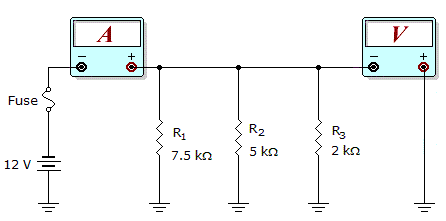# Electronics - Parallel Circuits - Discussion

Discussion Forum : Parallel Circuits - General Questions (Q.No. 28)
28.What would these meter readings indicate about the circuit in the given circuit?

Meter Readings: I = 10 mA, V = 12 V

R1 is open.
R2 is open.
The fuse is open.
The circuit is operating normally.
Explanation:
No answer description is available. Let's discuss.
Discussion:
8 comments Page 1 of 1.

Mohan rasu said:   9 years ago
Here, v=12v, r1=7.5 ohm, r2=5 ohm, r3=2 ohm.

WKT,
I=V/R.
I1=V/R1=12/7.5=1.6 mA.
I2=V/R2=12/5=2.4 mA.
I3=V/R3=12/2=6 mA.
Itot=10 mA.

So the ckt is operate normally.

Ash said:   10 years ago
How would you know that if r1 is open then c/n flow will be 8.4?

Waqar ahmad said:   10 years ago
Case 1.

When R1 is open then current flow in the circuit will be 8.4. Which is not equal to 10. So it is not correct.

Case 2.

When R2 is open then the current flow in the circuit will be 7.6 which is not correct as 10.

Case 3.

When fuse is open, the current and voltage would be zero.

Hence the circuit will operate normally.

Sunil Swarnkar said:   1 decade ago
Current through R1 = V/R1
= 12/7.5K = 1.6 mA

Current through R2 = V/R2
= 12/5K = 2.4 mA

Current through R3 = V/R3
= 12/2K = 6 mA

Total current 1.6+2.4+6 = 10mA

Ammeter is also showing 10mA.

So circuit is working normally.

Sreeyush Sudhakaran said:   1 decade ago
It=10mA
Vt=12v
Rt=Vt/It=12/10mA =1.2K

1/R1+1/R2+1/R3=1/1.2K

(1/7.5K)+(1/5K)+(1/2K)=0.833K (ALL K cancles)

0.133+0.2+0.5=0.833 is correct so none is open

circuit is operating normally

rt=0.00083
1/rt=1204.8129
after that,
it=vt/rt
so,
it=12/1204.8129
=9.96ma